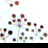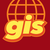# SHAPE@XY value are not centroids, instead they are truecentroids.

1025
4
11-29-2018 08:08 AMNew Contributor III

I am trying to calculate the centroids of polygons and found SHAPE@XY value are not centroids, instead they are truecentroids.

Script which I have used is.

lstFieldsList =  ["ID", "SHAPE@XY", "SHAPE@TRUECENTROID"]

with arcpy.da.SearchCursor(fc, lstFieldsList) as searchRows:

for searchRow in searchRows:

print searchRow,searchRow,searchRow[2

Result:

120 (13.397784197052292, 52.472906875319225) (13.397784197052292, 52.472906875319225)

I have found the workaround to extract centriods by using SHAPE@centroid.X, SHAPE@centroid.Y.

Shape file is attached for your test.

Is this a bug or I am doing something wrong?

I am using ArcGIS 10.6.1.9270 on Windows Server 2008_DE with service pack 1

Tags (2)
4 RepliesbyMVP Esteemed Contributor

Definitely a bug, I am seeing the same thing in Pro, but I am also seeing SHAPE@X and SHAPE@Y returning true centroid coordinates.

``````>>> import arcpy
>>>
>>> shape = arcpy.FromWKT(
...     'POLYGON((0 0, 100 0, 100 20, 20 20, 20 80, 100 80, 100 100, 0 100, 0 0))',
...     arcpy.SpatialReference(3857)
... )
>>> shape.centroid
<Point (50.0, 10.0, #, #)>
>>>
>>> shape.trueCentroid
<Point (40.76923076923077, 50.0, #, #)>
>>>
>>> fc = arcpy.CopyFeatures_management(shape, "in_memory/fc")
>>> flds = ["SHAPE@", "SHAPE@XY", "SHAPE@X", "SHAPE@Y", "SHAPE@TRUECENTROID"]
>>> with arcpy.da.SearchCursor(fc, flds) as cur:
...     for shape, shape_xy, shape_x, shape_y, shape_true in cur:
...         print("From shape:")
...         print("Geometry centroid: {}".format(shape.centroid))
...         print("Geometry true centroid: {}".format(shape.trueCentroid))
...         print("\nFrom Cursor:")
...         print("SHAPE@XY: {}".format(shape_xy))
...         print("SHAPE@TRUECENTROID: {}".format(shape_true))
...         print("SHAPE@X,SHAPE@Y: {}, {}".format(shape_x,shape_y))
...
...
From shape:
Geometry centroid: 50 10 NaN NaN
Geometry true centroid: 40.7692307692308 50 NaN NaN

From Cursor:
SHAPE@XY: (40.76923076923077, 50.0)
SHAPE@TRUECENTROID: (40.76923076923077, 50.0)
SHAPE@X,SHAPE@Y: 40.76923076923077, 50.0
>>> ‍‍‍‍‍‍‍‍‍‍‍‍‍‍‍‍‍‍‍‍‍‍‍‍‍‍‍‍‍‍‍‍‍‍``````byMVP Esteemed Contributor

Joshua... assuming those values are planar coordinates and not decimal degrees...

numpy/solution for the capital 'C'

_center(cw)
array([55., 50.])
_centroid(cw)  # uses the Shoelace formula to determine area first
array([40.77, 50.  ])
both as expected, of course non are expected to be in the C itself. (I had to clockwise the coordinates first)New Contributor IIby